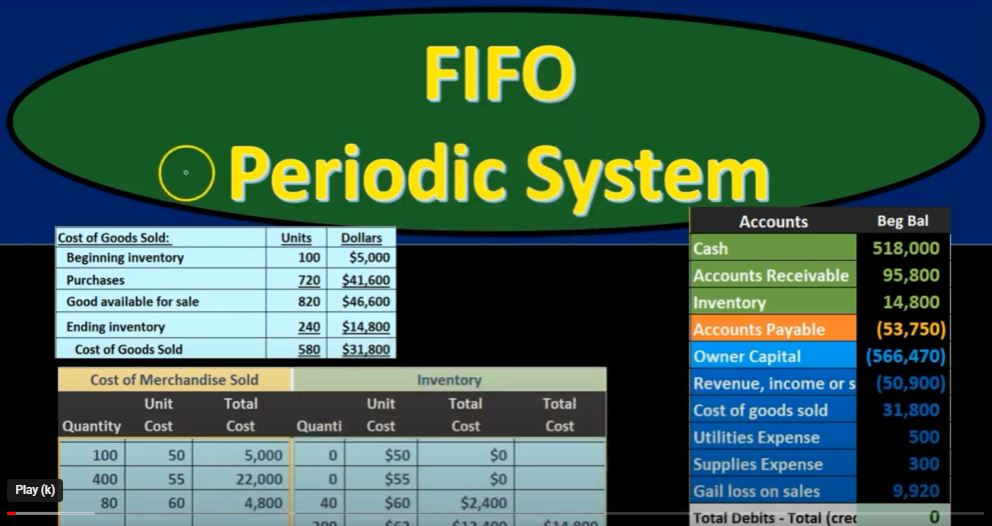## First In First Out (FIFO) Periodic System 42

In this presentation, we will delve into the concept of First In, First Out (FIFO) inventory valuation, comparing it within the context of both periodic and perpetual inventory systems. FIFO is one of the most widely used methods for valuing inventory, and understanding how it operates under different systems can be crucial for effective inventory management.

Introduction to FIFO

Before we proceed, let’s briefly explain what FIFO is. FIFO stands for First In, First Out, and it’s a method used to determine the value of inventory. Essentially, it assumes that the items that are added to inventory first are the ones that are sold first. In other words, the oldest inventory items are considered to be sold before the newer ones.

Now, let’s explore how FIFO operates within both periodic and perpetual inventory systems.

Periodic Inventory System

In a periodic inventory system, companies do not keep a real-time, item-by-item record of their inventory levels. Instead, they perform physical counts of inventory at specific intervals (usually at the end of an accounting period) to determine what’s on hand. This method is often employed by smaller businesses or those dealing with a limited range of products.

Under FIFO in a periodic system, the focus is mainly on recording the purchases throughout the period and the ending inventory. The cost of goods sold (COGS) is determined only at the end of the accounting period, making it an estimate of the cost of goods sold during that time.

Setting Up the Worksheet

To understand FIFO in a periodic system, it’s helpful to set up a worksheet. This worksheet typically consists of three main columns or sections: Purchases, Cost of Merchandise Sold, and Inventory. Each of these sections contains three components: quantity, unit cost, and total cost.

Here’s a breakdown of the sections:

1. Purchases: This section records all the inventory purchases made during the accounting period, including the quantity, unit cost, and total cost of each purchase.
2. Cost of Merchandise Sold: This section remains mostly unused during the accounting period under a periodic system. It becomes significant only at the end of the period when you calculate the final COGS.
3. Inventory: In this section, you maintain the record of your inventory, including the quantity, unit cost, and total cost. The ending inventory here will be used in the COGS calculation at the end of the period.

Starting with Beginning Inventory

In the example problem, we start with a beginning inventory of 100 units at a cost of \$50 each, totaling \$5,000. This is what we had on hand from previous periods.

Recording Purchases

Next, we record purchases made during the accounting period. For instance, on March 5, we purchased 400 units at \$55 each, totaling \$22,000. This purchase is documented in the Purchases section.

Completing the Worksheet

We continue to record purchases as they occur, updating the inventory section with the new additions. However, the Cost of Merchandise Sold section remains dormant throughout the period. This is because, in a periodic system, the actual calculation of COGS is postponed until the end of the accounting period.

Sales Transactions

During the period, we may also make sales transactions, but these only affect the Sales Revenue account and do not impact the inventory or COGS directly. The COGS calculation remains pending until the end of the period.

Final COGS Calculation

At the end of the accounting period (March in this case), we perform a physical count of our inventory. This count helps us determine the quantities on hand. In this example, we found that we had 240 units left.

Now comes the critical part: calculating the COGS for the period. The formula for COGS in a periodic system is as follows:

COGS = Beginning Inventory + Purchases – Ending Inventory

We apply this formula as follows:

• Beginning Inventory: \$5,000
• Purchases: \$22,000 + \$7,200 + \$12,400 = \$41,600
• Ending Inventory: \$14,800

COGS = \$5,000 + \$41,600 – \$14,800 = \$31,800

This \$31,800 is the estimated cost of goods sold for the period, which will be expensed on the income statement. Remember, this value represents an estimate because it assumes that we sold the oldest inventory items first.

Journal Entry

Finally, we make the journal entry to recognize the cost of goods sold:

• Debit: Cost of Goods Sold (Expense) – \$31,800
• Credit: Inventory (Asset) – \$31,800

This entry records the expense on the income statement, reducing the inventory on the balance sheet, and aligning our financial records with the physical count of inventory.

Conclusion

In a periodic inventory system, FIFO helps businesses estimate their cost of goods sold at the end of an accounting period. While it provides a reasonable approximation, it may not reflect the exact cost of goods sold during the period. Therefore, businesses often prefer perpetual inventory systems that maintain real-time inventory records for greater accuracy